# Bihar Board Class 9 Science Solutions Chapter 11 Work and Energy

## BSEB Bihar Board Class 9 Science Solutions Chapter 11 Work and Energy

Bihar Board Class 9 Science Solutions Chapter 11 Work and Energy Textbook Questions and Answers, Additional Important Questions, Notes.

### Bihar Board Class 9 Science Chapter 11 Work and Energy InText Questions and Answers

Page 148

Question 1.
A force of 7 N acts on an object. The displacent is, say 8 m, in the direction of the force (as given in the figure). Let us take it that the force acts on the object through the displacement. What is the work done in this case ?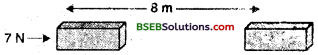Force, F = 7 N
Displacement, S = 8 m
Work, W = F x S
W = 7 N x 8 m = 56 Nm

Page 149

Question 1.
When do we say that work is done V Ans. Work is said to be done when force is applied on an object and the object moves through a distance in the direction of application of force. It is equal to the product of force and displacement. Work done = Force x Displacement or W = F x S

Question 2.
Write an expression for the work done when a force is acting on an object in the direction of its displacement.
When a force F is applied on a body, and it moves the body by a distance S in its direction, then the amount of work done is described by the relationship, Work done = Force x Distance t hrough which the body moves or, W = FxS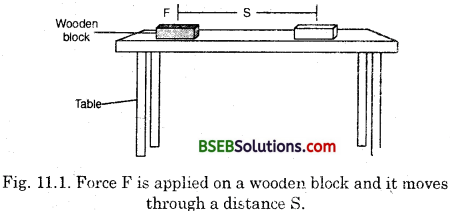Question 3.
Define 1 J of work.
Work done is described as,
Work = Force x Distance
So, the unit of work depends upon the unit of force and distance. In SI units, force is expressed in newton (N) unit, while the distance is measured in metre (m) unit. So,
1 unit of work done = 1 Newton x 1 Metre
The unit, 1 N x 1 m is called joule (J).
Thus, the SI unit of work is joule (J).
Joule is defined as follows :
The amount of work done when a force of 1 N moves a body by a distance of 1 m in its own direction is equal to 1 joule.Question 4.
A pair of bullocks exerts a force of 140 N on a plough. The field being ploughed is 15 m long. How much work is done in ploughing the length of the field ?
Force, F = 140 N
Displacements = 15 m
Work done = F x S
W = 140 Nx 15 m x
W = 2100 Nm
W = 2100J

Page 152

Question 1.
What is the kinetic energy of an object ?
The energy possessed by a Body by virtue of its motion is’called kinetic energy^So a moving object can do some work due to its kinetic energy.
Examples :
(i) A speeding bullet has kinetic energy.
(ii) Wind has kinetic,energy. It is due to this energy that tlie wind can turn the blades of a windmill.
(in) Flowing river water has kinetic energy.

Question 2.
Write an expression for the kinetic energy of an object.
Let a body of mass ‘in’ starting from rest accelerate uniformly to attain a velocity v after having covered a distance of s. Then,
v2 – u2 = 2as
or, u2 = 2as (u = 0)
a = $$\frac{v^{2}}{2 s}$$
So, the force (F) acting on the body is,
F = m x a = m x $$\frac{v^{2}}{2 s}$$
or, F x s = $$\frac {1}{2}$$ mv2
But, Fxs = Work done on the body
So,
Work done on the body = $$\frac {1}{2}$$ mv2
Kinetic energy of the body when it is in motion is equal to amount of work done on it. Therefore,
Kinetic energy of the body = $$\frac {1}{2}$$ mv2Question 3.
The kinetic energy of an object of mass, m moving with a velocity of 5 ms-2is 25 J. What will be its kinetic energy when its’ velocity is doubled ? What will be its kinetic energy when its velocity is increased three times ?
Mass = m2
Velocity, u = 5m-1
K.E. = 25 J
KE = $$\frac {1}{2}$$ m1v2
⇒ 25 = $$\frac {1}{2}$$ m1(5 ms-1)
⇒ 2 kg = m1

Case I : Velocity, u1 = 10 ms-1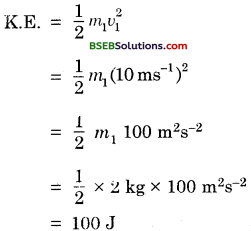Thus, when velocity is doubled, kinetic energy becomes four times.

Case II : Velocity, v2 = 15 ms-1Thus, when velocity is increased three times, its kinetic energy becomes nine times.

Page 156

Question 1.
What is power ?
Power is the rate of doing work or the rate of utilizing energy. The power of an agent may vary with time.

Question 2.
Define 1 watt of power.
1 watt is the power of an agent, which does work at the rate of 1 joule per second.
Or
Power is 1 watt when the rate of consumption of energy is 1 joule per second,

Question 3.
A lamp consumes 1000 J of electrical energy in 10 seconds. What is its power ?
Electrical energy = 1000 J
Time = 10 secondsP = 100 Js-1 or 100 W

Question 4.
Define average power.
We obtain the average power by dividing the energy consumed by the total time taken.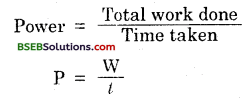### Bihar Board Class 9 Science Chapter 11 Work and Energy Textbook Questions and Answers

Question 1.
Look at the activities listed below. Reason out whether or not work is done in the light of your understanding of the term ‘work’.
(а) Suma is swimming in a pond.
(b) A donkey is carrying a load on its back.
(c) A wind-mill is lifting water from a well.
(d) A green plant is carrying out photosynthesis.
(e) An engine is pulling a train.
(f) Foodgrains are getting dried in the sun.
(g) A sailboat is moving due to wind energy.
(a) Yes, work is being done in this case. But the work done is negative since the force is being applied backwards but the displacement (motion of Suma) is in the forward direction.
(b) No, work is being done in this case because the force (load on its back) is acting downwards whereas the displacement (motion of the donkey) is in forward direction. For work to be done, displacement should be in the direction of application of force.
(c) Yes, work is being done in this case because the force is being applied upwards and the water is also being lifted upward.
(d) No, work is being done in this case because neither there is any force applied nor there is any displacement.
(e) Yes, work is being done because the engine is applying a force on the train and the train is showing displacement in the direction of force (moving forward).
(f) No, work is being done in this case because neither there is any force applied nor there is any displacement.
(g) Yes, work is being done in this case because the wind energy is applying a force and the sailboat is getting displaced in the direction of application of force.Question 2.
An object thrown at a certain angle to the ground moves in a curved path and falls back to the ground. The initial and the final points of the path of the object lie on the same horizontal line. What is the work done by the force of gravity on the object ? •
The work done by the force of gravity on the object will be zero. This is because,
Work done against gravity = m x g x h
The mass and acceleration due to gravity remains constant but the height becomes zero since the initial and final points of the path of the object lie on the same horizontal line.

Question 3.
A battery lights a bulb. Describe the energy changes involved in the process.
The chemical energy of the battery is converted into electrical energy which is further converted into heat and light energies.

Question 4.
Certain force acting on a 20 kg mass changes its velocity from 5 ms-1 to 2 ms-1. Calculate the work done by the force.
Mass, m = 20 kg
Initial velocity, u = 5 ms-1
Final velocity, v = 2 ms-1
Time, t = 1 s,
By first equation of motion,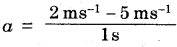By third equation of motion, v2 — u2 = 2as
img
Work done = F x s
W = m x a x s
W = 20 kg x (- 3 ms-2) x $$\frac {7}{2}$$m
W = – 210 Joules

Question 5.
A mass of 10 kg is at a point A on a table. It is moved to a point B. If the line joining A and B is horizontal, what is the wrork done on the object by the gravitational force ? Explain your answer.
Mass, m = 10 kg Acceleration due to gravity, g = 10 ms-2
Work done by force of gravity = m x g x h
In this case, the work done on the object by the force of gravity is zero because the line joining A and B is horizontal, hence the height ‘h’ is zero, i.e., there is no component of the force of gravity in the direction of displacement.

Question 6.
The potential energy of a freely falling object decreases progressively. Does this violate the law of conservation of energy ? Why?
This does not violate the law of conservation of energy because as the potential energy of the freely falling object decreases, its kinetic energy increases. Hence, the total energy remains the same.

Question 7.
What are the various energy transformations that occur when you are riding a bicycle ?
The various energy transformations are Potential energy to muscular energy to mechanical energy.Question 8.
Does the transfer of energy take place when you push a huge rock with all your might and fail to move it ? Where is the energy you spend going?
No, transfer of energy does not take place when we push a huge rock with all our might. When we push the rock, the muscles are stretched and blood is displaced to the strained muscles more rapidly. These changes consume energy and we feel tired.

Question 9.
A certain household has consumed 250 units of energy during a month. How much energy is this in jpules ?
Energy consumption during a month = 250 units
1 unit = 1 kWh
250 units = 250 kWh
Now l kWh = 36,00,000 J
250 kWh = 36,00,000 J x 250
= 90,00,00,000 J

Question 10.
An object of mass 40 kg is raised to a height of 5 m above the ground. What is its potential energy? If the object is allowed to fall, find its kinetic energy when it is half-way down.
Mass, m = 40 kg
Height, h = 5m
Acceleration due to gravity, g = 10 ms-2
P.E. = m x g x h
= 40 kg x 10 ms-2 x 5 m = 2000 J

When the object is half-way down
Mass, m = 40 kg
Height, h = 2.5 m
Acceleration due to gravity,
g = 10 ms-2
Initial velocity, u = 0 ms-1
By third equation of motion,
v2 – u2 = 2as
or v2 = u2+ 2as
or v2 = 0 + 2 x 10 ms-2 x 2.5 m
= 20 ms-2
K.E. = $$\frac {1}{2}$$ mv2
K.E. = $$\frac {1}{2}$$ x 40 kg x 20 x 2.5 m2 s-2
K.E. = 1000 J

Question 11.
What is the work done by the force of’ gravity on a satellite moving round the earth ? Justify your answer.
The work done on a satellite moving round the earth is zero. When the satellite moves in a circular path, then the centripetal force acts towards the centre along the radius, and the direction of motion is tangential to the circle. The two are thus perpendicular to each other (Fig. 12.12). So,
Work done = F x S x cos θ
W = FS cos 90° = 0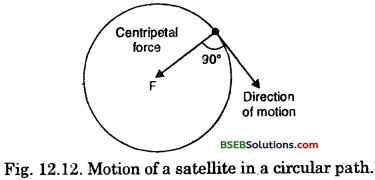So, in the. case of uniform circular motion, the work done is zero.

Question 12.
Can there be displacement of an object in the absence of any force acting on it ? Think. Discuss this question with your friends and teacher.
When the body is at rest, by Newton’s first law of motion, it continues to remain at rest unless an external unbalanced fprce acts on it. Hence, no displacement takes place in the absence of force.
However, when the body is in motion, say a moving bus, a force is required to stop it. So, in this case, the displacement takes place in the absence of force.Question 13.
A person holds a bundle of hay over his head for 30 minutes and gets tired. Has he done some work or not ? Justify your answer.
No, the person has not done any work on the bundle of hay because there is no displacement of the bundle.

Question 14.
An electric heater is rated 1500 W. How much energy does it use in 10 hours ?
Power = 1500 W = $$\frac {1500}{1000}$$kW = 1.5kW
Time = 10hElectrical energy = Power x Time taken
Electrical energy 1.5 kW x 10 h = 15 kWh

Question 15.
Illustrate the law of conservation of energy by discussing the energy changes which occur when we draw a pendulum bob to one side and allow it to oscillate. Why does the bob eventually one to’ rest ? What happens to its energy eventually ? Is it a violation of the law of cnservation of energy?
(i) The bob eventually comes to rest because the friction at the point of support of the pendulum and friction of air acting on the swinging bob converts the mechanical energy of the oscillating pendulum into heat energy slowly.
(ii) Eventually this heat energy goes into the environment.
(iv) No, it is not a violation of the law of conservation of energy.

Question 16.
An object of mass, m is moving with a constant velocity, v. How much work should be done on the object in order to bring the object to rest ?
Mass = m
Constant velocity = υ
Energy of the body = mυ2 (since the body is in motion)
Thus, an equivalent amount of work should be done on the body in order to bring it to rest.
Hence work done on the object = $$\frac {1}{2}$$ mυ2

Qusestion 17.
Calculate the work required to be done to stop a car of 1500 kg moving at a velocity of 60 km/h ?
Mass, m = 1500 kg
Velocity, v = 60 kmh-1
= $$\frac{60 \times 1000 \mathrm{~m}}{3600 \mathrm{~s}}$$ = 16.67 ms-1
K.E. = $$\frac {1}{2}$$ mv2
K.E. = $$\frac {1}{2}$$ x 1500 kg x (16.67 ms-1)2 = 208416.67 JQuestion 18.
In each of the following a force ‘F’ is acting on an object of mass, m. The direction of displacement is from west to east shown by the longer arrow. Observe the diagrams carefully and state whether the work done by the force F is negative, positive or zero.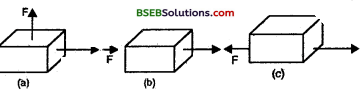(a) In this case, the work done is zero since the force is acting perpendicular to the displacement.
Work done at an angle 9 = F x s x cos θ
∴ W = F x s x cos 90°
W = F x s x 0 = 0 Joule
(b) In this case, the work done is positive because the.
displacement takes place in the direction of application of force.
(c) In this casey the work done is negative because the displacement takes place in the direction opposite to the direction of application of force.

Question 19.
Soni says that the acceleration in an object could be zero even when several forces are acting on it. Do you agree with her ? Why ?
Yes, Soni is correct because when the object is – at rest,, the velocity is zero and in turn the acceleration
is zero. Several forces may act on it but they cancel each other. When the object is in motion and moving with a constant velocity, its acceleration is zero. Even in this situation,, several forces may act on the object which balance each other.

Question 20.
Find the energy in kWh consumed in 10 hours by four devices of power 500 W each.
Power of each device = 500 W
Power of four devices = 4 x 500 W = 2000 W = $$\frac {2000}{1000}$$ KW = 2KW
Time = 10 hours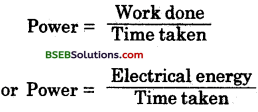Electrical energy = Power x Time taken
Electrical energy = 2 kW x 10 hrs = 20 kWh

Question 21.
A freely falling object eventually stops on reaching the ground. What happens to its kinetic energy ?
When-the object stops since the velocity becomes
zero, its kinetic energy also becomes zero (K.E. = $$\frac {1}{2}$$ mv2) on reaching the ground.

### Bihar Board Class 9 Science Chapter 11 Work and Energy Textbook Activities

Activity 11.1

Question 1.
A number of activities have been discussed on the page no. 147 in the text-book which we normally consider to be workin day-to-day life. For each of these activities, ask the following question and answer them:
(i) What is the work being done on ?
(ii) What is happening to the object ?
(iii) Who (what) is doing the work ?
(i) The work is being done on his mind and body.
(ii) The object (student) is getting exerted.
(iii) The student is doing work.

Activity 11.2

Question 1.
Think of some situations from your daily life involving work. List them. Discuss with your friends whether work is being done in each situation. Try to reason out your response.
(i) If work is done, which is the force acting on the object ?
(ii) What is the^object on which the work is done ?
(iii) What happens to the Object on which work is done ?
Case I : Pushing a table by a boy.
(i) The force acting on the object is the muscular force ot the boy.
(ii) The work is done on the table.
(iii) The object moves when the work is done. :

Case II: When a person carries a handbag and wa’ks on a level road.
(i) A muscular force is being applied by the person vertically upwards.
(ii) No work is being done because the motion is in horizontal direction and the force is applied vertically upwards. There is no displacement in the direction of application of force.Activity 11.3

Question 1.

• Think of situations whejn the? object is -not displaced in spite of a force acting on it.
• Also think of situations when’ an object gets displaced in the absence of a force acting on it.

(i) List all the situations that you can think of for each.
(ii) Discuss with your friends whether work is done in these situations.
(i) In the following situations the object is not displaced inspite of a force acting on it. .
(a) A man pushing a wall.
(b) A boy pushing a stationary truck.
(c) A girl carrying a book on her head and standing still. (Force of gravity acts on the book).
(ii) There can be no situation where displacement can be produced without action of any force. In fact, if there is displacement in an object, always there will be certain force acting on it.
(iii) Work is not done in these situations because two conditions need to be satisfied for work to be done.
(a) a force should act on an object and
(b) the object must be displaced.

Activity 11.4

Question 1.
Lift an object up. Work is done by the force exerted by you on the object. The object moves upward. The force you exerted is in the direction of displacement. However, there is the force of gravity acting on the object.
(i) Which one of these forces is doing positive work ?
(ii) Which one is doing negative work ? Give reasons.
(i) The force exerted by us is doing a positive work.
(ii) The force of gravity is doing a negative work. Work done is negative when the force acts opposite to the direction of displacement. Work done is positive when the force is in the direction of displacement. Positive work by a force increases the speed of the object while negative work decreases it.

Activity 11.5

Question 1.
The sun is the biggest natural source of energy to us. We can also get energy from the nuclei of atoms, the interior of the earth and the tides.
(i) A few sources of energy are listed above. There «re many other sources of energy. List them.
(ii) Discuss in small groups how certain sources of energy are due to the Sun.
(iii) Are there sources of energy which are not due to the Sun?
(i) (a) Potential energy
(b) Kinetic energy
(c) Mechanical energy
(d) Heat energy
(e) Chemical energy
(f) Electrical energy
(g) Light energy
(ii) (a) The air near the earth is heated by sun’s heat energy. The hot air becomes lighter (in density) and rises up creating a vacuum below. Simultaneously, cooler air (having higher density) flows in to take place of hot air. This flowing in air is wind. Thus, wind energy is indirectly derived from the solar energy.

(b) Fossil fuels (e.g. coal, petroleum, natural gas, etc.) are obtained from dead plants and animals. These plants and animals got buried below earth’s crust millions of years ago. Due to sun’s energy and high pressure, they got converted into fuels. Thus, energy of fossil fuels is derived indirectly from the sun.

(iii) Yes, there are sources of energy which are not due to the sun. For example, nuclear energy, chemical energy, electrical energy etc.Activity 11.6

Question 1.
Take a heavy ball. Drop it on a thick bed of sand. A wet bed of sand would be better. Drop the ball on the sand bed from a height of aboutT2lTciTE The ball creates a depression. Repeat this activity from heights of 50 cm, 1 m and 1,5 m. Ensure that all the depressions are distinctly visible. Mark the depressions to indicate the height from which the ball was dropped. Compare their depth.
(i) Which one of them is deepest ?
(ii) Which one is shallowest ? WTiy ?
(iii) What has caused the ball to make a deeper dent?
(i) Deepest depression is created when the ball is dropped from a height of 1.5 m.
(ii) Shallowest depression is created when the ball is dropped from a height of 25 cm.
This is because when the ball is dropped from a greater height it has more energy in it as it does more work.
(iii) The high velocity and the high energy has caused the ball to make a deeper dent.

Activity 11.7

Question 1.
Set up the apparatus as shown in the figure 11.6. Place a wooden block of certain known mass in front of the trolley at a convenient fixed distance. Place a known mass on the pan so that the trolley starts moving.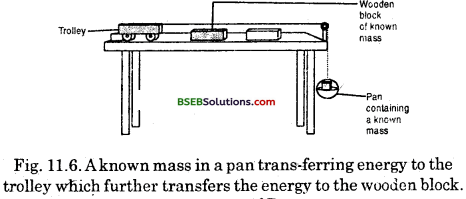The trolley moves forward and hits the wooden block. The block gets displaced.
(i) What does this mean ?
(ii) From where does the block gain energy ?
(iii) Repeat this activity by increasing the mass on the pan. In which case is the displacement more? In which case is the work done more?
(iv) Which formula can Tbe used to calculate the work done ?
(i) This means work is done on the block as it has gained energy..
(ii) The block gains energy from the wooden block kept in the trolley. When a known mass of wooden block is kept in a pan, it moves down and, as a result, pulls the trolley alongwith the wooden block. In turn, tjie wooden block in the trolley transfers its energy to the block.
(iii) In the second case the displacement and the work done will be more.
(iv) The work done can be calculated by using the
formula W = F x S
or W = m x g x sActivity 11.8

• Take a rubber band. Hold it at one end and pull the other.

(i) What happens to the band ?
(ii) What happens when’you release the band ?
(iii) Did the band acquire any energy in the stretched position ? How did it acquire this energy ?
(i) When we hold the rubber band on one end and pull the other, the band is stretched.
(ii) When we release the band, it moves back to its original position and regains its original shape.
(iii) Yes, the band acquired energy in the stretched position. When we pull the band, we apply a force on the band which does work on the band. This work in turn transfers energy to the band. The energy transferred to the band is stored as potential energy. This energy is equal to the work done by us in stretching the band.

Activity 11.9

• Take a slinky as shown below. Ask a friend to hold one of its ends. You hold the other end and move away from your friend. Now you release the slinky.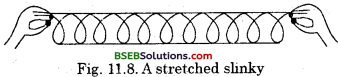(i) What happened ?
(ii) How did the slinky acquire energy when stretched ?
(iii) Would the slinky acquire energy when it is compressed ?
(i) When we release the slinky it will go back towards our friend.
(ii) When we stretch the slinky we apply a force on it which does work on the slinky. This work in turn transfers energy .to the slinky.
(iii) Yes, the slinky will have some energy even when it is compressed.

Activity 11.10

• Take a toy car  Wind it using its key. Place the car on the ground.

(i) Did it move ?
(ii) From where did it acquire energy ?
(iii) Does the energy acquired depend on the number of times you turn the key ? How can you test this?
(i) Yes, the car moved.
(ii) We do work while winding the key of the toy car. The energy is transferred to the spring inside the toy and is stored as potential energy. This energy is equal to the work done by us in winding the toy.
(iii) Yes, the energy acquired depends on the number of times we turn the key. The more we turn the key the greater will be the energy acquired. We can test this by turning the key for less number of times initially and placing the toy on the ground. Then we can turn the key for more number of times and place the toy on the ground. We will observe that the car moves for a longer time when we turn the key more number of times.Activity 11.11

• Lift an object through a certain height. The object now can do work.

(i) What happens to the object when it is released ? What does it imply ?
(ii) What happens to the object at higher positions ? From where did it get the energy ?
(i) The object begins to fall when it is released. This implies that it has acquired some energy.
(ii) At higher positions, the object can do more work and hence possesses more.energy. We apply force in lifting the object. As a result, we do work on the object. The energy is transferred to the object which is stored as potential energy. This energy is equal to the work done by us in lifting the object.

Activity 11.12

• Take a bamboo stick and make a bow as shown in Fig. 11.9. Place on arrow made of a light stick on it with one end supported by the stretched string.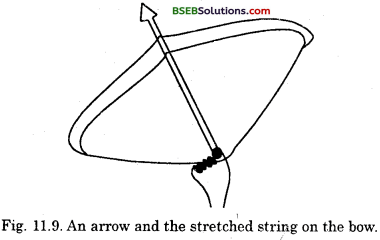(i) What will happen if the string is stretched and the arrow is released ?
(ii) What will happen to the shape of the bow ?
(iii) How is the arrow thrown off from the bow ?
(i) If the string is stretched and the arrow is released, the arrow will fly off the bow.
(ii) The shape of the bow Will be changed. It will become straight once the arrow is released.
(iii) The potential energy stored m the bow due to the change of shape us used in the form of kinetic energy in throwing off tlie arrow.Activity 11.13

• Sit in small groups.
• Discuss the various ways of energy conversion in nature.
• Discuss following questions in your group :

(a) How do green plants produce food?
(b) Where do they get their energy from?
(c) Why doesTthe air move from place to place?
(d) How are fuels, istich as coal and petroleum formed?
(e) What kinds of energy conversion^ sustain
the water cycle ?
(a) Green plants produce food by the process of photosynthesis.
(b) They get their energy from the sun.
(c) The air near the earth is heated by sun’s heat energy. The hot air becomes lighter (in density) and rises up creating a vacuum below. Simultaneously, cooler air (having higher density) flows in to take place of hot air. This flowing in air is wind. Thus, wind energy is indirectly derived from the solar energy.
(d) Fossil fuels (e.g. coal, petroleum, natural gas, etc.) are obtained from dead plants and animals. These plants and animals got buried below earths crust millions of years ago. Due to sun’s energy and high pressure, they got converted into fuels. Thus, energy of fossil fuels is derived indirectly from the sun.
(e) Solar energy to chemical energy7.

Activity 11.14

• Many of the human activities and the gadgets we use involve conversion of energy from one form to another.

(i) Make a list ‘of such activities and gadgets.
(ii) Identify in each activity/gadget the kind of energy conversion that takes place.
(i) Some human activities and gadgets involving conversion of energy are :
(а) An arrow released from a bow
(b) Electric fan
(c) Electric heater
(d) A stone falling from a building
(e) Pendulum bob is released from one of the extreme position to mean position ,
(f) A waterfall
(g) Winding of spring of a watch
(h) Bus moving up a hill (0 Steam engine
(i) Electric motor
(k) Microphone
(l) Loudspeaker(ii) The energy transformation in the above activities/ gadgets are as follows :
(а) Potential to kinetic
(b) Electric to mechanical
(c) Electric to heat
(d) Potential to kinetic
(e) Potential to kinetic
(f) Potential to kinetic
(g) Potential, to kinetic
(h) Kinetic to potential
(i) Heat to mechanical
(j) Electric to mechanical
(k) Electric to sound
(l) Sound to electrical

Activity 11.15

Question 1.
An object of mass 20 kg is dropped from a height of 4 m. Compute the potential energy and kinetic energy when the object is located at a height of 4 m, 3 m, 2 m, 1 m and just above the ground. [g = 10 ms-2]
Mass of the body, m = 20 kg
Height, h = 4 m
g = 10 ms-2
When the mass is at the top
P.E. = m x gx h = 20 kg x 10 ms-2 x 4 m = 800 J
K.E, = $$\frac {π}{2}$$ mv2
= $$\frac {π}{2}$$ x 20 kg x {0 ms-1)2 = 0 J

When the mass is at a distance
When the body starts failing, it gains kinetic energy and loses a part of the potential energy. Using the third
equation of motion, v2 – u2 = 2as, where s is the distance travelled by the falling body. v2 = u2 + 2as
v2 = 0 + 2 x 10 ms2 x s
v2 = 20 s ms-2
K.E. = $$\frac {1}{2}$$ mυ2
P.E. mg (h – s)
P.E. = 20 kg x 10 ms-2 x (4 m – s)

When the body is at a height of 3 m from the ground
h = 3 m
s = 4 m – 3 m = 1 m
∴ K.E. = $$\frac {1}{2}$$mυ2
K.E. = $$\frac {1}{2}$$ x 20 kg x 20 x 1 m2s-2
K.E. = 200 J
P.E. = m x g x (4 m – s)
P.E. = 20 kg x 10 ms-2 x (4 m – 1 m)
P.E. = 600 J
The calculated values of P.E. and K.E. at various stages of fall are given in the table given on the next page.Thus, energy is conserved during a free fall.

Activity 11.16

Question 1.
Consider two children, say A and B. Let us say they weigh the same. Both start climbing up a rope separately. Both reach a height of 8 m. Let us say A takes 15 seconds while B takes 20 seconds to accomplish the task.
(i) What is the work done by each.?
(ii) Is the work done same ?
(iii) Who has done more work in a given time, say, 1 second ? How ?
(i) Let the mass of each child -in Acceleration due to gravity,
g = 10 ms-2
Height, h = 8 m
Work done, W = F x s
W = m x g x h
W = m x 10 x 8
W = 80 m Joule
(ii) Yes, the work doiie is the same.
(iii) A has done more work in 1 second.
Work done by A in 15 s = 80 m Joule
Work done by A in 1 s =$$\frac {80m}{15}$$ Joule = 5.33 m Joule
Work done by B in 20 s = 80 m Joule
Work done by B in 1 s = $$\frac {80m}{20}$$ Joule = 4m JouleActivity 11.17

• Take a close look at the electric meter installed in your house. Observe its features closely.
• Take the readings of the meter each day at 6.30 am and 6.30 pm.

(i) How many ‘units’ are consumed during day time?
(ii) How many ‘units’ are used during night?
(iii) Do this activity for about a week.
(v) Draw inferences from the data.
(vi) Compare your observations with the details given in the monthly electricity bill.
Do it yourself. (The observation may vary from house to house.)

### Bihar Board Class 9 Science Chapter 11 Work and Energy Additional Important Questions and Answers

Question 1.
Name the physical quantities which are closely related to work.
Energy and power.

Question 2.
What do you mean by life processes ?
The basic activities performed by living beings for. their survival are known as life processes.

Question 3.
From where do we get energy for the life processes ?
The energy from the life processes comes from food.

Question 4.
Name some activities other than the life * processes for which we need energy.
We need energy for all kinds of activities like reading, writing, playing, singing, jumping, running, etc.

Question 1.
Push a pebble lying bn a surface such that it moves through a distance.
(i) What happened to the pebble ?
(ii) What made the pebble move ?
(iii) Is work being done scientifically ?
(i) The pebble got displaced.
(ii) The force exerted on the pebble made it move.
(iii) Yes, work is being done scientifically because force is applied and the pebble is displaced.

Question 2.
Jennifer pulls a trolley and the trolley moves through a distance.
(i) What happens to the trolley ? Why ?
(ii) Is work being done scientifically ?
(i) The trolley is displaced because Jennifer has exerted-a force on it.
(ii) Yes, work is being done scientifically because force is applied and the trolley is displaced.

Question 1.
Prove that the energy remains constant in the ease of freely falling bodies.
Consider an object of mass m, at rest at a height h above the surface of ground.
Potential energy at A = mgh
Kinetic energy are A = 0 ‘
Total energy at A = mgh + 0 = mgh
Now, the object falls freely. After travelling a distance x, it reaches at the point B. At this point, its height above the ground is (h – x). Then,
Potential energy at B = mg (h – x)
Initial velocity at A = 0
If v is the final velocity at B,
then v2= u2 + 2gxi.e., v2 = 2 gx (v u = 0)
Then, kinetic energy at B
= $$\frac {1}{2}$$ mv2
= $$\frac {1}{2}$$ x m x 2 gx = mgx

Total energy at B
= mg (h – x) + mgx
= mgh – mgx + mgx
= mgh
Now, the object reaches at C after travelling a distance h. For this motion, u = 0 .
If v is the final velocity, at C,
then v2 = u2 + 2 gh
= 0 + 2 gh = 2 gh
Kinetic energy at C = $$\frac {1}{2}$$ mv2
= $$\frac {1}{2}$$ m x 2 gh = mgh and,
Potential energy at C = mg x 0 = 0
So,
Total energy at, C = mgh + 0 = mgh
Thus, the total energy of the object at the position A
lts oual to the total energy at the positions B and C.
Then a freely falling body has the same energy at everjr point during its motion.